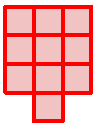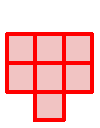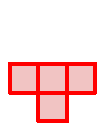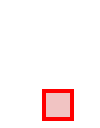### Home > CCAA8 > Chapter 2 Unit 2 > Lesson CCA: 2.1.3 > Problem2-34

2-34.

Draw Figures 1, 2, and 3 for a tile pattern that could be described by $y=−3x+10$.

Use figure zero as a starting point. The growth is $−3$, so remove three tiles for the next figure.Figure 0Figure 1Figure 2Figure 3

Use the eTool below to create a pattern that could be described by $y=−3x+10$.
Click the link to the right to view full version. 2-34 HW eTool (CPM)